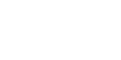Construction:
1) draw to the fold of the fabric perpendicular, on which lay off 1/2 of the mid-svaga value (1) - AA1
A1A2 = 7 - 10 cm (for processing)
AA2 = 0,20 + 0,10 = 0,30 m
2) fold delay value (3) - the depth of the Smad with k (2)
AB = 0.45 x 2 = 0.90 m
3) BV = 1/2 value (3) = 0,45 : 2 = 0.23 m
4) From point B hold perpendicular to the bend
5) From point B are arc equal to the value of the lower of the SAG (4) is obtained by measuring the perpendicular drawn from the point B
VG = total (4) + 5 cm (for processing) = 85 + 5 = 0.90 m
6) Connecting the points A2 and d, the resulting value (2) - Smad with a shoulder k on the depth of laying folds.